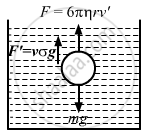Advertisement Remove all ads

# A Metal Sphere of Radius 1 Mm and Mass 50 Mg Falls Vertically in Glycerine. Find (A) the Viscous Force Exerted by the Glycerine on the Sphere When the Speed of the Sphere is 1 Cm S−1, - Physics

Answer in Brief

A metal sphere of radius 1 mm and mass 50 mg falls vertically in glycerine. Find (a) the viscous force exerted by the glycerine on the sphere when the speed of the sphere is 1 cm s1, (b) the hydrostatic force exerted by the glycerine on the sphere and (c) the terminal velocity with which the sphere will move down without acceleration. Density of glycerine = 1260 kg m−3 and its coefficient of viscosity at room temperature = 8.0 poise.

Advertisement Remove all ads

#### Solution

Given:
Radius of metallic sphere r = 1 mm = 10−3 m
Speed of the sphere v = 10−2 m/s
Coefficient of viscosity η = 8 poise = 0.8 decapoise
Mass m = 50 mg = 50 × 10−3 kg
Density of glycerin σ = 1260 kg/m3

(a) Viscous force exerted by glycerine on the sphere F = 6πηrv
⇒ F= 6 × (3.14) × (0.8) × 10−3 × (10−2)
= 1.50 × 10−4 N
(b) Let V be the volume of the sphere.
Hydrostatic force exerted by glycerin on the sphere

$F' = V\sigma g$

$\Rightarrow F' = \frac{4}{3}\pi r^2 \sigma g$

$= \left( \frac{4}{3} \right) \times \left( 3 . 14 \right) \times \left( {10}^{- 6} \right) \times 1260 \times 10$

$= 5 . 275 \times {10}^{- 5} \text{N}$

(c) Let the terminal velocity of the sphere be v'.
The forces acting on the drops are
(i) The weight mg acting downwards
(ii) The force of buoyance, i.e.,  $\frac{4}{3}\pi r^3 \sigma g$  acting upwards

(iii) The force of viscosity, i.e., 6πηrv' acting upwards
From the free body diagram:$6\pi\eta r v' + \frac{4}{3}\pi r^3 \sigma g = \text{ mg}$

$\Rightarrow v = \frac{\text{ mg }- \frac{4}{3}\pi r^2 \sigma g}{6\pi\eta r}$

$= \frac{50 \times {10}^{- 3} - \frac{4}{3} \times 3 . 14 \times {10}^{- 6} \times 1260 \times 10}{6 \times 3 . 14 \times 0 . 8 \times {10}^{- 3}}$

$= \frac{500 - \frac{4}{3} \times 3 . 14 \times {10}^{- 3} \times 1260 \times 10}{6 \times 3 . 14 \times 0 . 8}$

$= 2 . 3 \text{ cm/s }$

Concept: Viscosity
Is there an error in this question or solution?
Advertisement Remove all ads

#### APPEARS IN

HC Verma Class 11, 12 Concepts of Physics 1
Chapter 14 Some Mechanical Properties of Matter
Q 30 | Page 301
Advertisement Remove all ads

#### Video TutorialsVIEW ALL 

Advertisement Remove all ads
Share
Notifications

View all notifications

Forgot password?